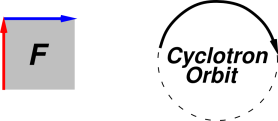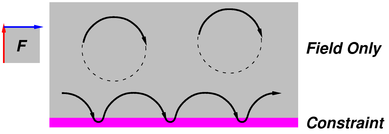[Contents]

## 1  Background

By way of background, suppose we have a magnetic field that is uniform in space. Let’s consider the motion of a charged particle in this field. In this section, we are dealing with a “free” particle, meaning that it is not subject to any forces other than those arising from the our aforementioned magnetic field.

Our magnetic field can be represented by a bivector in the plane of the paper, as shown in figure 1. This is equivalent to saying the old-fashioned magnetic field vector is perpendicular to the paper, directed out of the paper. For more on this, see reference 1.Figure 1: Lorentz Law

In the case where the initial velocity of the particle is in the plane of the paper, the motion is a circle, called a cyclotron orbit, as shown in the figure.

More generally, if the particle has a component of velocity perpendicular to the paper, this component is unaffected by the magnetic field, and can be considered separately. The most general motion is a helix: circular motion of the in-plane components and uniform linear motion of the out-of-plane component.

For simplicity, we will have nothing further to say about the out-of-plane component.

## 2  Current in a Wire in a Magnetic Field

Now let’s see what happens if our particle is constrained to remain inside a wire. In figure 2, the wire runs left-to-right; we are seeing a small section of a long straight wire. If the particle is deep in the interior of the wire, it moves in a cyclotron orbit as previously discussed. There is no way to get such particles to carry any macroscopic current along the wire.Figure 2: Current in a Wire in a Field

However, some of the particles will be within one orbit-diameter of the surface of the wire. There is some non-magnetic force that keeps the particles inside the wire. For simplicity, we model this event as more-or-less simple reflection. The wire exerts a force on the particles, and by the same token the particles exert a force on the wire. (Remember that we have taken the viewpoint that the particles are mostly-free particles, moving freely inside the wire unless/until they smack into the force of constraint. They are not “attached” to the wire except insofar as the constraint makes them so.)

Also we see that there is a net left-to-right drift of the particles near the surface of the wire. This is how the wire carries a macroscopic current.

## 3  Discussion

There is a famous theorem that says that in a frame where the electromagnetic field is purely magnetic, the field by itself cannot do any work on a test charge. The proof is super-easy: the magnetic force is always perpendicular to the velocity. The power (i.e. the rate of work) is force dot velocity. The dot product is zero, because the vectors are perpendicular. That’s all there is to it.

There is a similar theorem that says a static constraint does no work.

However, as we have seen, a magnetic field can exert a force on a current-carrying wire, and if the wire moves, work will be done on the wire. (You can build an electromagnetic railgun using this principle.)

There is no contradiction here; a static constraint does no work, but for a moving constraint, all bets are off. For example, water is held in a ladle by forces of constraint, but you can easily do work on water, ladling it from a lower bucket to a higher bucket.

It is a fool’s errand to argue about whether the force on the wire is “because” of the magnetic force on the particles, or “because” of the force of constraint. You need both the magnetic force and the force of constraint to explain the observed phenomena. (For more about cause-and-effect and its relevance – or lack thereof – to the force laws, see reference 2.)

Remember that we have been discussing positively-charged particles. You can easily re-derive the results in terms of negatively-charged particles if you wish.

## 4  References

1.
John Denker, “Electromagnetism using Geometric Algebra versus Components” ./maxwell-ga.htm

2.
John Denker, “Cause and Effect” ./causation.htm
[Contents]